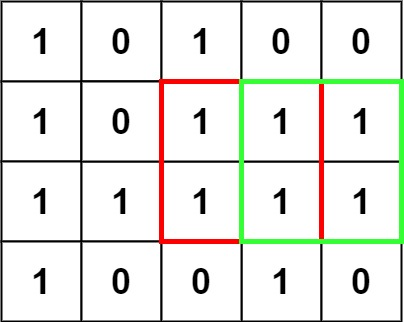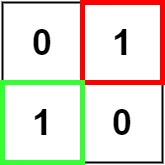# 221. Maximal Square | LeetCode Solution

## 221. Maximal Square | LeetCode Solution

Given an `m x n` binary `matrix` filled with `0`'s and `1`'s, find the largest square containing only `1`'s and return its area.

Example 1:```Input: matrix = [["1","0","1","0","0"],["1","0","1","1","1"],["1","1","1","1","1"],["1","0","0","1","0"]]
Output: 4
```

Example 2:```Input: matrix = [["0","1"],["1","0"]]
Output: 1
```

Example 3:

```Input: matrix = [["0"]]
Output: 0
```

Constraints:

• `m == matrix.length`
• `n == matrix[i].length`
• `1 <= m, n <= 300`
• `matrix[i][j]` is `'0'` or `'1'`.

class Solution {
public:
int maximalSquare(vector<vector<char>>& v) {
int m=v.size();
int n=v.size();
int ans=0;
vector<vector<int>>dp(m,vector<int>(n,0));
for(int i=0; i<m; i++){
if(v[i][n-1]=='1'){dp[i][n-1]=1;ans=1;}
}
for(int i=0; i<n; i++){
if(v[m-1][i]=='1'){dp[m-1][i]=1;ans=1;}
}

for(int i=m-2; i>=0; i--){
for(int j=n-2; j>=0; j--){
if(v[i][j]=='1'){
dp[i][j]=1+min(dp[i+1][j],min(dp[i+1][j+1],dp[i][j+1]));
ans=max(ans,dp[i][j]);
}
}
}
return ans*ans;

}
};